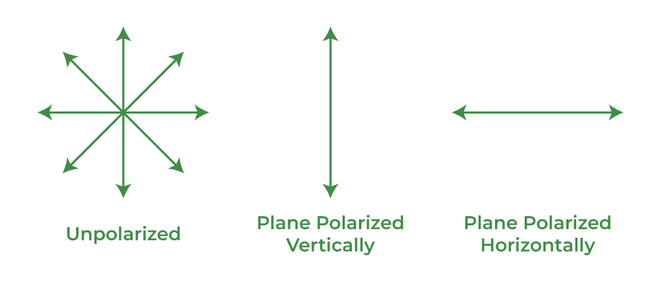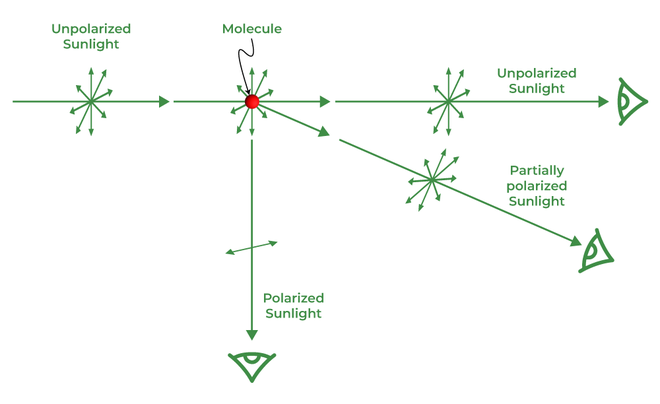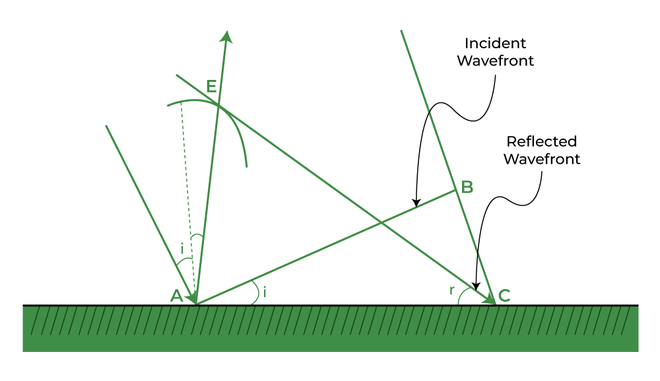Open in App
Not now

# Polarisation by Scattering and Reflection

• Last Updated : 22 Aug, 2022

Physical optics, frequently known as wave optics, is a discipline of optics that looks at obstruction, diffraction, polarization, and different peculiarities for which the mathematical optics beam estimate isn’t material. The connection between waves and light beams is very much portrayed by wave optics. Light is a sort of energy that moves through a medium as a cross-over wave, as per the wave hypothesis of light. At some random time, the wavefront is the area of all particles vibrating in only a similar recurrence. To finish up, a wavefront is a surface on which all vibrating particles have practically a similar recurrence at any one point.

## Theory of Wave Optics

The field of wave optics has seen a prestigious discussion between two huge logical social orders committed to concentrating on the idea of light. One society accepts that light is a molecule, while the other accepts it is a wave. Both beam optics and wave optics are unimaginably significant as they depend on the rectilinear spread of light and manage mirrors, focal points, reflection, refraction, and so on.

Sir Isaac Newton is a notable ally of the molecule idea of light, proposing a corpuscular hypothesis where “light comprises of very light and little particles known as corpuscles that movement at incredibly high rates from the wellspring of light to drum up some excitement of vision by considering the retina of the eye.” Newton had the option to depict reflection and refraction utilizing this hypothesis, yet he couldn’t make sense of the reasons for impedance, diffraction, and polarization. The principal imperfection in Newton’s corpuscular hypothesis was that it neglected to make sense of why the speed of light in denser media was lower than in a vacuum. Then, Huygens concocted his hypothesis in designing material science of wave optics.

## Polarization

If we get out of the home on a hot radiant day, we will utilize shades. Likewise, if we are watching a 3D film, we would utilize 3D glasses. In the two cases, polarization assumes a vital part. Let’s see what polarization is and concentrate on it exhaustively. Polarization is only changing unpolarised light into spellbound light. Unpolarised light is the light wherein particles vibrate in all kinds of planes.

### Ways of Polarizing lightIn the above figure, we see that there is a plane of vibration parrel to the plane. Likewise, there is a plane of vibration opposite to the plane. Over, the principal picture is of an unpolarised one. Polaroids are polarizing materials comprising particles adjusted in a specific course. Each Polaroid has a pass pivot. It will permit light to go just through the pass pivot. A polaroid can have a level pass pivot as well as an upward pass hub. These decide how the light will go through it. So when an unpolarized light goes through a polaroid, it gets energized.

## Polarization by Scattering

At the point when light episodes on a particle or an iota, it retains the light energy and yet again produce it every which way. This dispersing is because of polarization. Additionally, the radiated light goes this way and that. Besides, we get dissipated light when unpolarized light is an episode on a molecule. So when unpolarized light goes through a particle, it energizes the light toward the path opposite to the occurrence beam. Accordingly, the polarization of light occurs toward this path.

Furthermore, this is the way polarization happens by dissipating light. The dispersed light is toward a path opposite the heading of the episode beam. Additionally, polarization is finished in dispersed light while it is halfway in light going through atoms.In the above figure, we can see the episode beam, reflected beam, and refracted beam. On the episode beam, we see the unpolarized light. The unpolarized light is meant as displayed in the above figure. Here the speck addresses opposite bearings, and lines demonstrate the equal course. It is seen that the vast majority of the light in the reflected beam is energized and lined up with the plane with not many unpolarised ones. While in the refracted beam, a large portion of the light is unpolarised with a couple of spellbound parts. In this manner, we see that both the reflected and the refracted beam are, to some degree, spellbound.

## Polarization by Dichroism

At the point when light is an episode on a piece of tourmaline gem, it parts into two captivated refracted beams because of two-fold refraction. Out of 2 refracted beams, one is conventional, and the other is uncommon/In conventional beams, the vibrations opposite to the chief segment of the gem are consumed by the gem, and the uncommon beam has vibrations lined up with the chief part of the precious stone communicated through the gem. The new beam is plane-captivated with a yellow-green tone. This peculiarity of specific ingestion of the gem is known as dichroism.
The polaroid depends on this guideline.

### Brewster’s Law

The law expresses that the reflected beam is enraptured at a specific point of rate. Additionally, the point between the reflected beam and refracted beam is 90°. On the off chance that I = iB, for example, at the point when the point of rate is equivalent to Brewster’s point, then complete point = 90°.

Then by Snell’s law, we say that,

Sini / Sinr = μ

∠iB + r  =  90°

r = 90°- iB

⇒ tan iB = μ

## Reflection of Waves

As we are probably aware, when light falls on an article, it twists and travels through the material; this is the very thing refraction is. Likewise, when the light bobs off the medium, it is known as a reflection. Tell us to concentrate on all things being equal and the refraction of waves by Huygen’s rule.

### Huygens’ Wave Theory

Nobody had scrutinized Newton’s corpuscular hypothesis until Christopher Huygens concocted his hypothesis of wave optics in designing physical science in the mid-eighteenth hundred years. Light, as indicated by Huygens, is a wave. He envisioned a wave peak pushing ahead by imagining each point along the peak as a source point for nearly nothing, roundabout, extending wavelets that grow at a similar rate as the wave. The shape of the propelling is still up in the air by the surface digression of these wavelets. Huygens’ making of reflection and refraction is portrayed in the graph beneath.

According to Huygens’ principle,

1. Every point on a wavefront produces optional wavelets and works as a light source.
2. With the speed of light, auxiliary wavelets spread every which way in space (vacuum).
3. The new place of the still up in the air by the envelope of optional wavelets along the forward course after a set period.

Light reflection, refraction, impedance, and diffraction were completely made sense of by Huygens’ wave optics hypothesis. Yet, he couldn’t make sense of Polarization since Huygens respected light waves to be longitudinal mechanical unsettling influences.
Compton Effect, photoelectric impact, and dark body radiation. The ether, a speculative medium that was rarely found, however, which we currently know can proliferate light in a vacuum.

### Reflection using Huygens’ principle

We can see a beam of light is an episode on this surface, and another beam that is lined up with this beam is likewise occurring on this surface. Plane AB is episode at a point ‘I’ on the reflecting surface MN. As these beams are episodes from the surface, so we call them occurrence beams. If we draw an opposite from point ‘A’ to this beam of light, Point A and point B will have a line going along with them, and this is called a wavefront, and this wavefront is an occurrence on a superficial level.This episode wavefront conveys two focuses, point An and point B, so we can express that from direct B toward point C, light is voyaging a distance. If ‘ v ‘ addresses the speed of the wave in the medium and if ‘ r ‘ addresses the time taken by the wavefront from the direct B toward C, then the distance,

BC = vr

Altogether, they develop the reflected wavefront. We draw a circle of range vr from point A. Allow CE to address the digression plane drawn from guide C toward this circle. In this way,

AE = BC = vr

Assuming we currently consider the triangles EAC and BAC, we will observe that they are harmonious; hence, the points ‘I’ and ‘r’ would be equivalent. This is the law of reflection.

## Solved Examples on Polarisation by scattering and Reflection

Example 1: A ray of light is incident on the surface of the plate of glass of refractive index 2 at the polarising angle. Then the angle of refraction of the ray will be,

1. 16.6°
2. 26.6°
3. 36.6°
4. 46.6°

Solution:

B. If iP be the polarising angle, then, tan  iP = μ = 2 or iP = 63.4°

The angle of refraction, r = 90° –  iP

= 90° – 63.4°

= 26.6°

Example 2: In the event of straightly spellbound light, the greatness of the electric field vector.

1. Changes intermittently with time.
2. It doesn’t change with time.
3. Increments or diminishes directly with time.
4. It is lined up with the bearing of engendering.

A. In a light whether enraptured or unpolarized, the greatness of the electric field vector generally shifts occasionally with time.

Example 3: The refractive index of the denser media is 1. Calculate the polarizing angle of the medium.

1.  60°
2.  30°
3.  45°
4.  90°

Solution:

C. According to Brewster’s law,

tan ip = n

Therefore polarizing angle ip = tan-1 n [Here n = 1]

= tan-1(1)

= 45°

Example 4: A ray of light strikes a glass plate at an angle of 45°. If the reflected and refracted rays are perpendicular to each other, the index of refraction of glass is

1.  2
2.  1
3.  3
4.  4

B.  As the refracted ray and reflected ray is perpendicular the angle of refraction is 45°

Apply snell’s law,

μ1 sinα12 sinα

1 × sin45° = μ × sin45°

μ = 1

Example 5: The angle of incidence at which the polarisation of light reflected from the surface of the glass is 50°, then the refractive index of glass is?

Solution:

Brewster’s angle is an angle of incidence at which light with a particular polarization is perfectly transmitted through a transparent dielectric surface, with no reflection.

The Brewster’s angle is given as,

50° = tan −1 (n1 / n2

50° = tan −1(μ / 1)

μ = tan50°

μ = 1.1918

Example 6: Monochromatic light of wavelength 985 nm is incident from the air on a water surface. What are the wavelength, frequency, and speed of reflected?

Solution:

The wavelength of incident monochromatic light,

= 985 nm = 985 × 10−9

Speed of light in air = 3 × 108 m/s

Refractive Index of water = 1.33

The reflected ray will reflect in the same medium as that of the incident ray medium. Hence, the wavelength, frequency, and speed of the reflected ray will be the same as that of the Incident ray because of the same medium.

The frequency of light is,

= 3 × 108/ 985 × 10−9

= 3.04 × 1014 Hz

Hence, the wavelength, frequency, and speed of the reflected light in the same medium are 985 nm, 3.04 × 1014 Hz, and 3 × 108 m/s respectively.

Example 7: A ray of light is incident on the surface of a glass plate of refractive index 4.55 at the polarizing angle. The angle of the refraction is approximate.

Solution:

The polarizing angle, or Brewster’s angle, is the angle at which an incident ray gets reflected and refracted through the medium such that the two beams are perpendicular to each other.

By Snell’s Law, n1 sin i = n2 sin r

Also i + r = 90°

Thus, n1 sin (90° – r) =n2 sin r

Hence, r= tan −1 (n1 / n2

= tan −1 (1 / 4.55)

### FAQs on Polarisation by scattering and Reflection

Question 1: What is polarized light used for?

• Polaroid sunglasses are used to reduce glare.
• A 3-D effect can be achieved by using polarized light.

Question 2: What do we mean when we say that the light from the sun is unpolarized?

Since the electric field produced by the light is distributed in all directions, after passing through the atmosphere, the light gets semi-polarized. In order for the sunlight to be polarized, the angle of incidence must be equal to the angle of polarization.

Question 3: What are the different types of polarization?